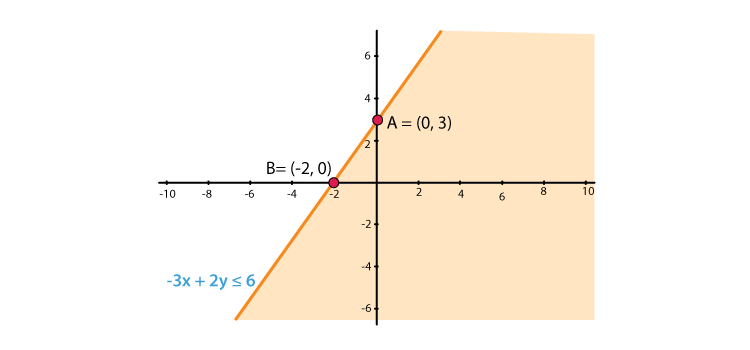# RD Sharma Solutions for Class 11 Chapter 15 - Linear Inequations Exercise 15.5

The graphical solution of linear inequations in two variables are discussed in this section. Students who find difficulty in solving problems can refer to RD Sharma Class 11 Maths Solutions for quick reference. Our expert tutors have solved the problems in a step by step manner so that each and every student can understand the methodology used, to solve the problems. Students are advised to prepare from RD Sharma textbook, which helps in securing high marks in their board exams. Students can download the pdf of RD Sharma Class 11 Solutions from the links given below.

## Download the pdf of RD Sharma Solutions for Class 11 Maths Exercise 15.5 Chapter 15 – Linear Inequations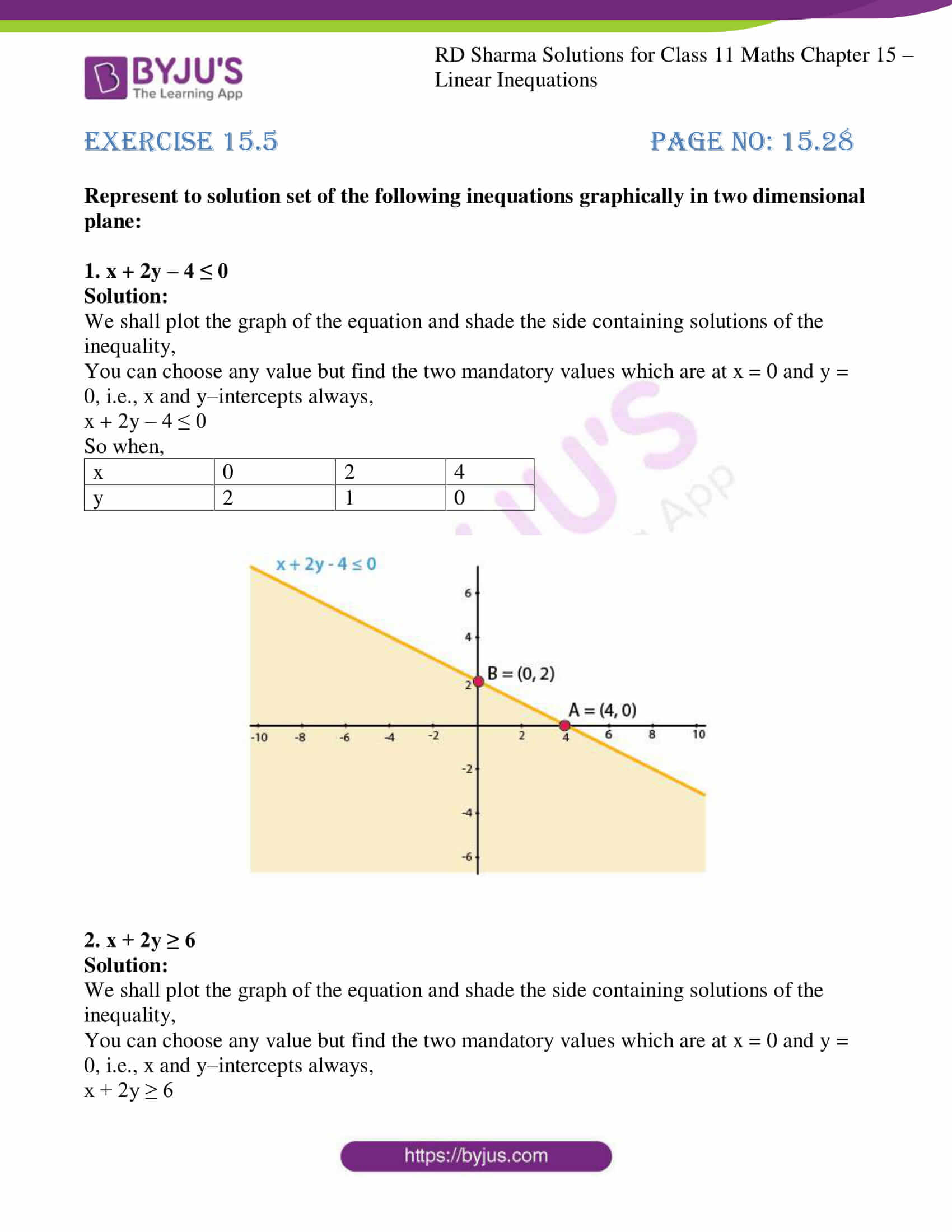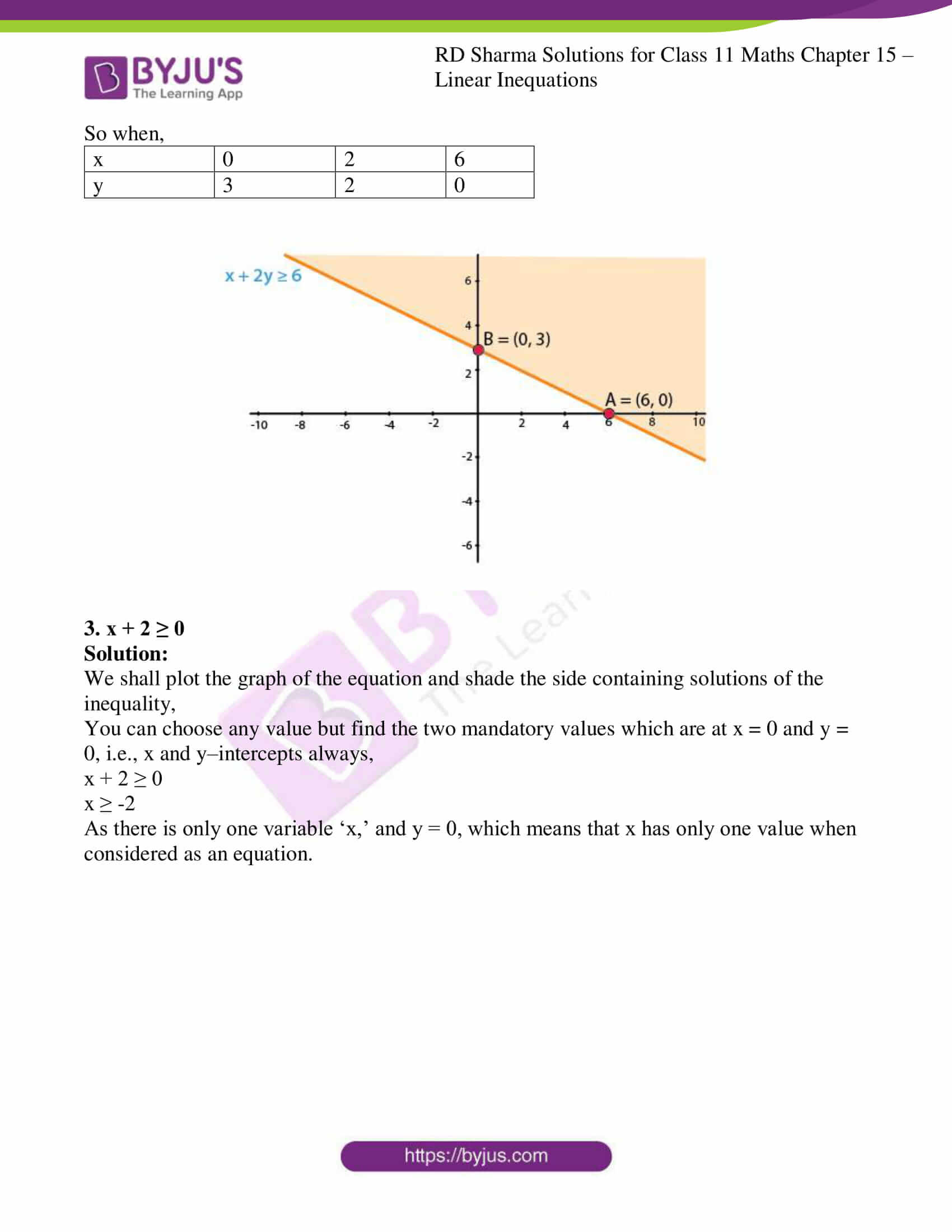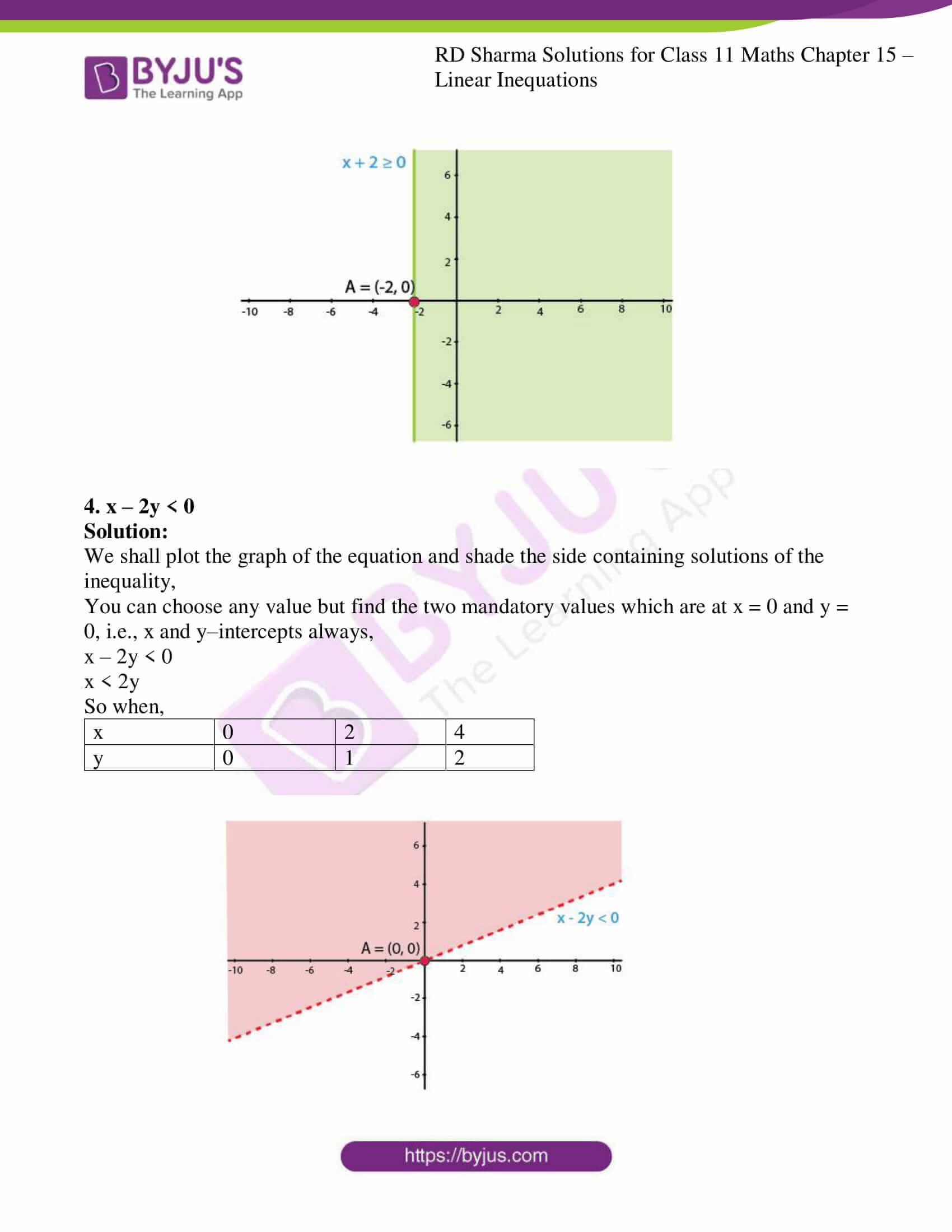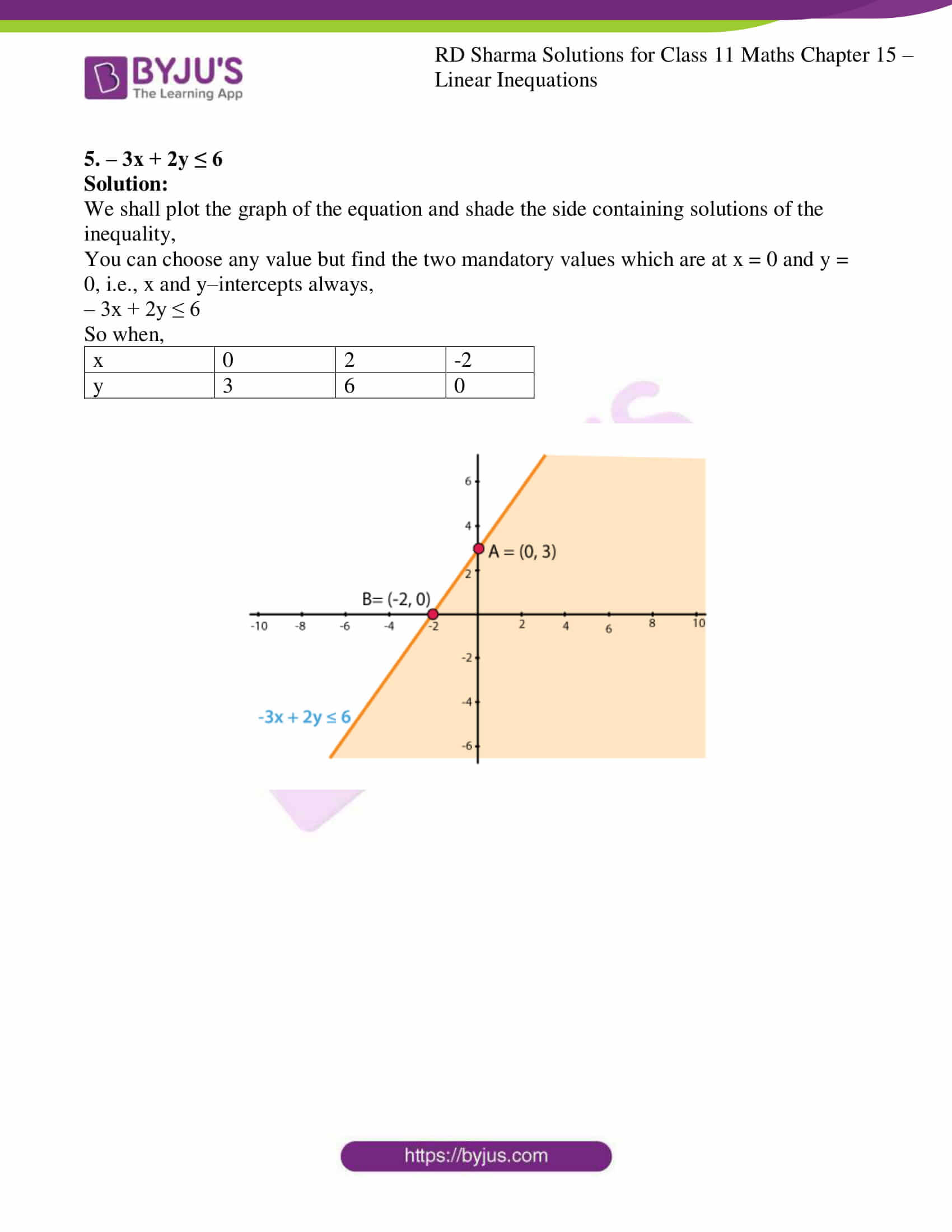### Access answers to RD Sharma Solutions for Class 11 Maths Exercise 15.5 Chapter 15 – Linear Inequations

Represent to solution set of the following inequations graphically in two dimensional plane:

1. x + 2y – 4 ≤ 0

Solution:

We shall plot the graph of the equation and shade the side containing solutions of the inequality,

You can choose any value but find the two mandatory values which are at x = 0 and y = 0, i.e., x and y–intercepts always,

x + 2y – 4 ≤ 0

So when,

 x 0 2 4 y 2 1 0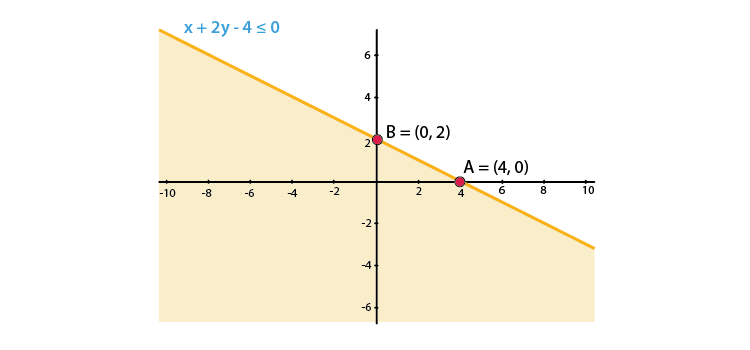2. x + 2y ≥ 6

Solution:

We shall plot the graph of the equation and shade the side containing solutions of the inequality,

You can choose any value but find the two mandatory values which are at x = 0 and y = 0, i.e., x and y–intercepts always,

x + 2y ≥ 6

So when,

 x 0 2 6 y 3 2 0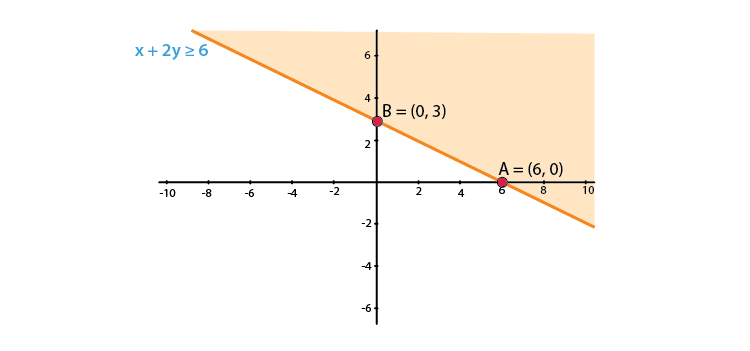3. x + 2 ≥ 0

Solution:

We shall plot the graph of the equation and shade the side containing solutions of the inequality,

You can choose any value but find the two mandatory values which are at x = 0 and y = 0, i.e., x and y–intercepts always,

x + 2 ≥ 0

x ≥ -2

As there is only one variable ‘x,’ and y = 0, which means that x has only one value when considered as an equation.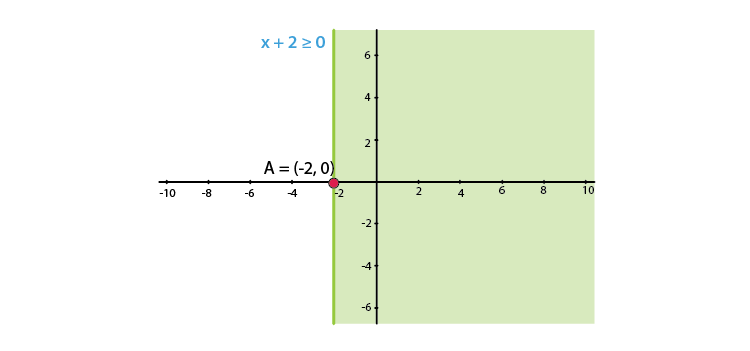4. x – 2y < 0

Solution:

We shall plot the graph of the equation and shade the side containing solutions of the inequality,

You can choose any value but find the two mandatory values which are at x = 0 and y = 0, i.e., x and y–intercepts always,

x – 2y < 0

x < 2y

So when,

 x 0 2 4 y 0 1 2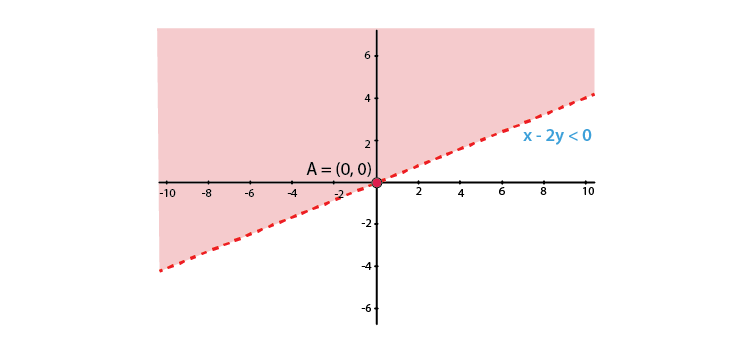5. – 3x + 2y ≤ 6

Solution:

We shall plot the graph of the equation and shade the side containing solutions of the inequality,

You can choose any value but find the two mandatory values which are at x = 0 and y = 0, i.e., x and y–intercepts always,

– 3x + 2y ≤ 6

So when,

 x 0 2 -2 y 3 6 0